# ResNet-101 for 3D Morphable Model Regression Trained onCasia WebFace Data

Represent a facial image as a vector

Released in 2016, this model takes a facial image as input and produces a 198-dimensional feature vector representing its 3D morphable model. The feature vector produced by the net is meant to be consumed by the Basel Face Model; the first half of the vector (the first 99 components) represents the 3D shape while the second represents texture. The model can be effectively used as a generic feature extractor.

Number of layers: 346 | Parameter count: 43,011,206 | Trained size: 174 MB |

## Training Set Information

• Casia WebFace, a collection of 494,414 facial photographs of 10,575 subjects.

## Performance

• When used as a face recognition system, the model acheives 88.8% of accuracy on the YouTube Faces dataset and 92.35% accuracy on the Labeled Faces in the Wild dataset.

## Examples

### Resource retrieval

Get the pre-trained net:

 In:=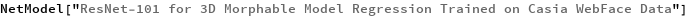Out=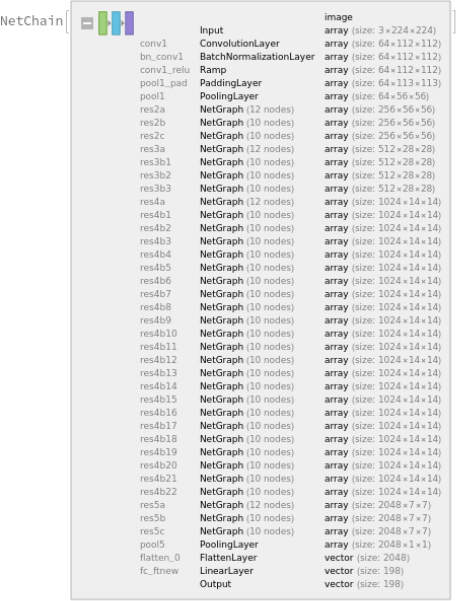### Basic usage

Compute a feature vector for a given image:

 In:=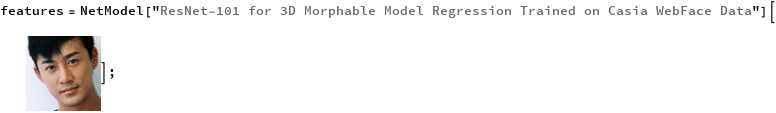Get the length of the feature vector:

 In:=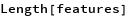Out=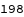Use a batch of face images:

 In:=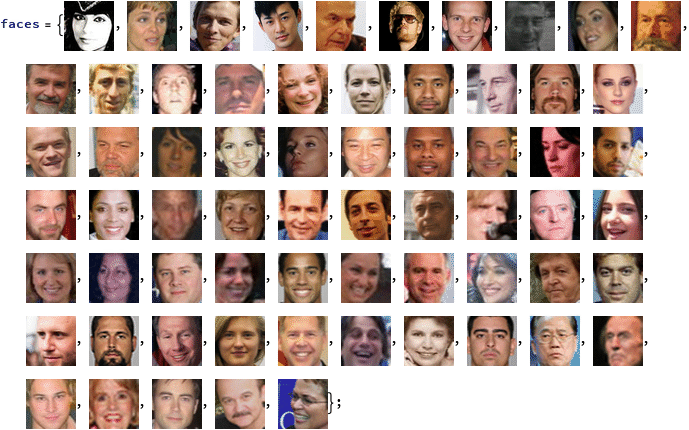Compute the feature vectors for the batch of images and obtain the dimensions of the features:

 In:=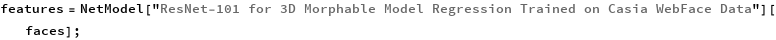In:=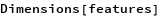Out=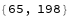Reduce the feature vectors to two dimensions with t-SNE:

 In:=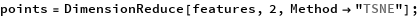In:=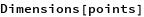Out=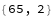Visualize the results:

 In:=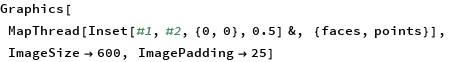Out=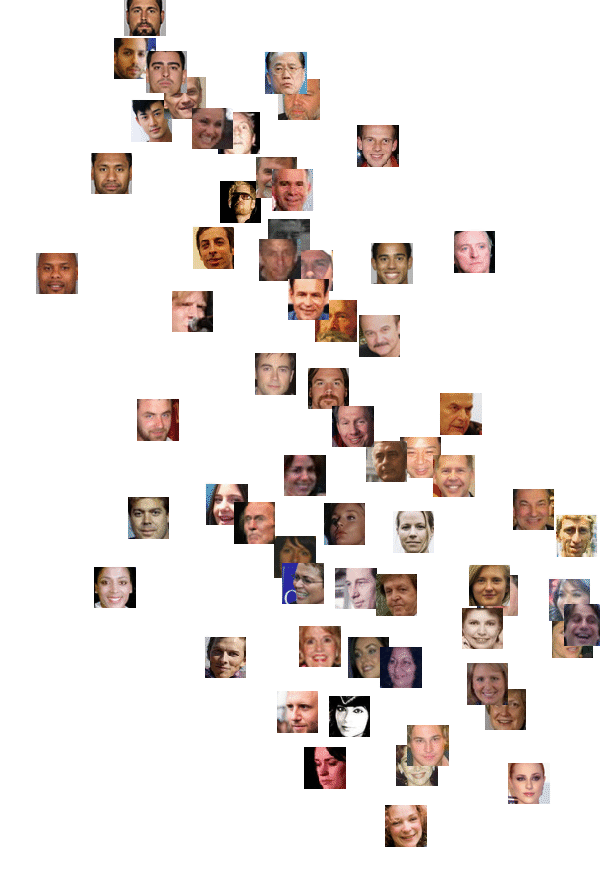Obtain the five closest faces to a given one:

 In:=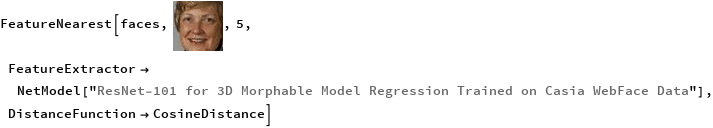Out=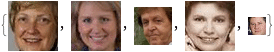### Net information

Inspect the number of parameters of all arrays in the net:

 In:=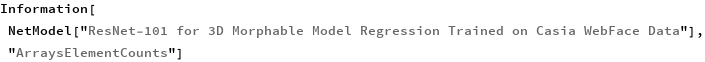Out=Obtain the total number of parameters:

 In:=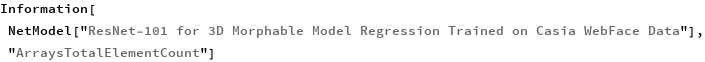Out=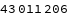Obtain the layer type counts:

 In:=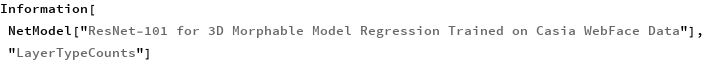Out=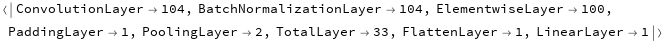Display the summary graphic:

 In:=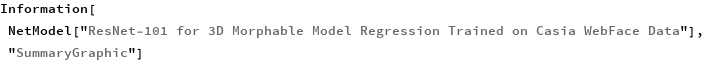Out=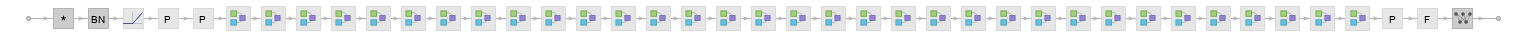### Export to MXNet

Export the net into a format that can be opened in MXNet:

 In:=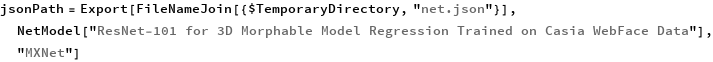Out=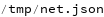Export also creates a net.params file containing parameters:

 In:=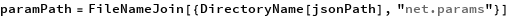Out=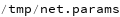Get the size of the parameter file:

 In:=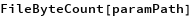Out=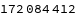The size is similar to the byte count of the resource object:

 In:=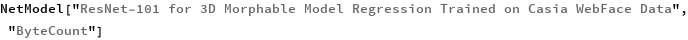Out=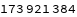## Requirements

Wolfram Language 12.1 (March 2020) or above